# Texas Go Math Kindergarten Lesson 13.5 Answer Key Doubles

Refer to our Texas Go Math Kindergarten Answer Key Pdf to score good marks in the exams. Test yourself by practicing the problems from Texas Go Math Kindergarten Lesson 13.5 Answer Key Doubles.

## Texas Go Math Kindergarten Lesson 13.5 Answer Key Doubles

Essential Question
How can you write addition sentences for doubles?

Explore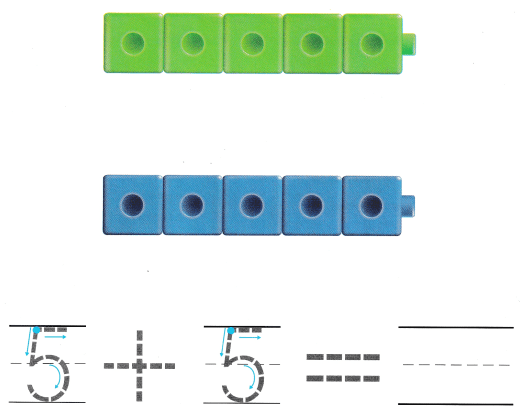Explanation:
2 groups of 5 cube trains
5 + 5 = 10
so, 10 cubes in all
The sum of 5 and 5 is 10.

Directions
Place green cubes on the pictured cubes. Trace the number. Place blue cubes on the pictured cubes below. Trace the number. Add the sets. Write the sum.

Share and Show

Question 1.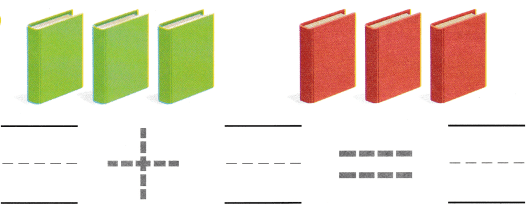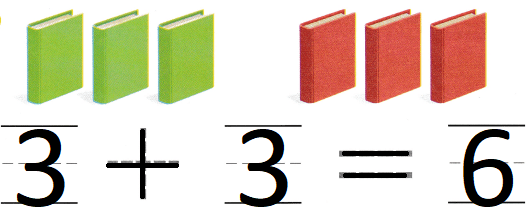Explanation:
2 groups of 3 books each
3 + 3 = 6
so, 6 books in all.
The sum of 3 and 3 is 6

Question 2.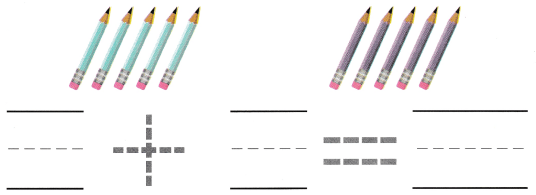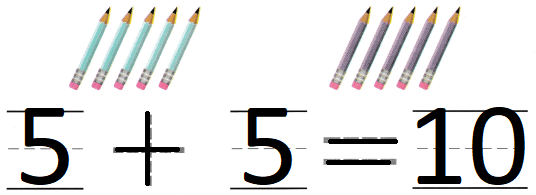Explanation:
2 groups of 5 pencils each.
5 + 5 = 10
The sum of 5 and 5 is 10
so, 10 pencils in all.

Question 3.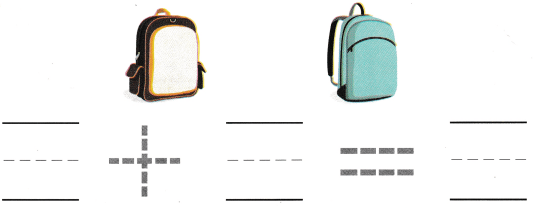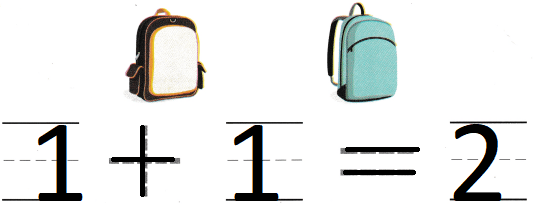Explanation:
The sum of 1 and 1 is 2
1 + 1 = 2
So, 2 bags in all.

Directions
1-3. Write the number in each group. Add. Write the sum. Trace the symbols.

Question 4.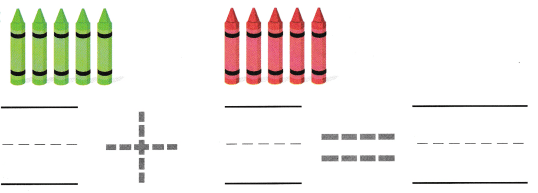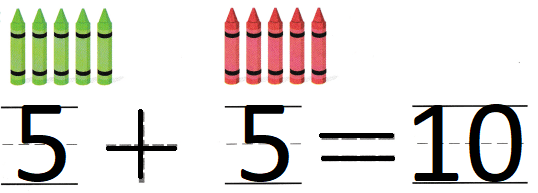Explanation:
2 groups of 5 crayons each
5 + 5 = 10
so, 10 crayons in all.
The sum of 5 and 5 is 10.

Question 5.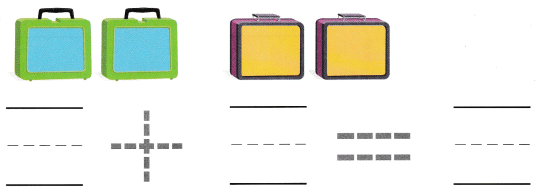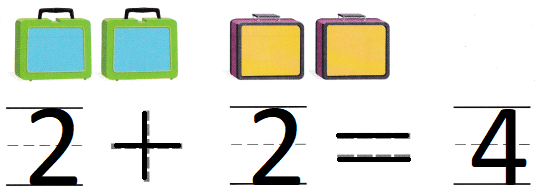Explanation:
2 sets of 2 bags each
2 + 2 = 4
so, 4 bags in all.
The sum of 2 and 2 is 4

Question 6.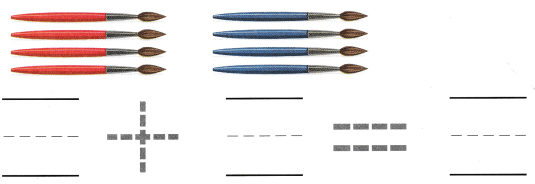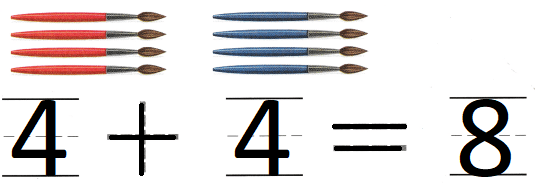Explanation:
2 groups of 4 painting brushes
4 + 4 = 8
so, 8 painting brushes in all.
The sum of 4 and 4 is 8.

Directions
4-6. Write the number in each group. Add. Write the sum, Trace the symbols.

Home Activity

• Show your child a set of 1 to 5 objects such as crayons. Have your child create a set of objects that shows the some number of objects and find the sum.

Problem Solving

Question 7.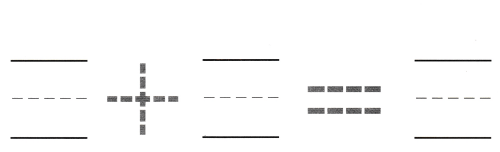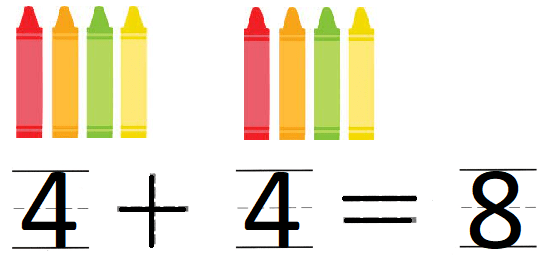Explanation:
2 groups of 4 crayons
4 + 4 = 8
The sum of 4 and 4 is 8

Question 8.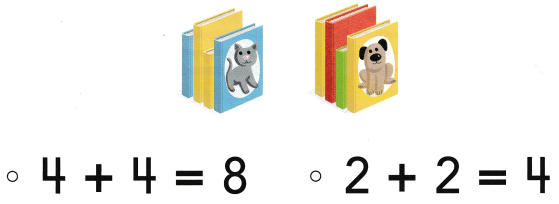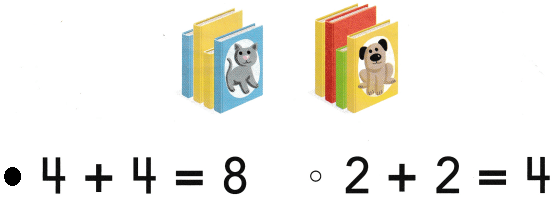Explanation:
2 groups of 4 books
4 + 4 = 8
so, 8 books in all.
The sum of 4 and 4 is 8

### Texas Go Math Kindergarten Lesson 13.5 Homework and Practice

Question 1.Explanation:
2 groups of 3 crayons
3 + 3 = 6
so, 6 crayons in all.
The sum of 3 and 3 is 6

Question 2.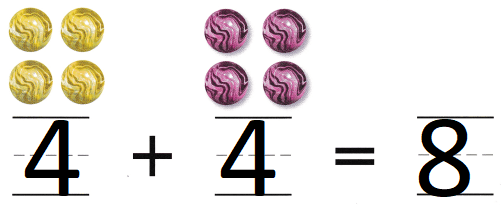Explanation:
2 groups of 4 marbles
4 + 4 = 8
so, 8 marbles in all
the sum of 4 and 4 is 8

Question 3.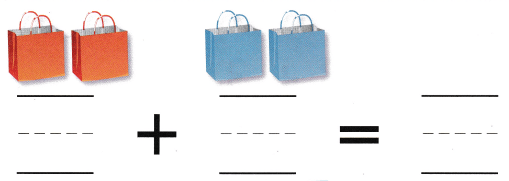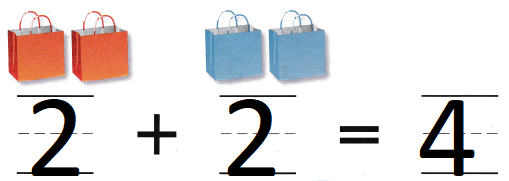Explanation:
2 groups of 2 bags
2 + 2 = 4
the sum of 2 and 2 is 4

Directions
1-3. Write the number in each group. Add. Write the sum

Texas Test Prep

Lesson Check

Question 4.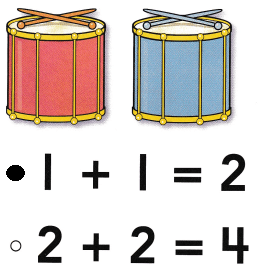Explanation:
The sum of 1 and 1 is 2
1 + 1 = 2

Question 5.